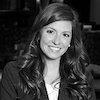AuthorTopic: t test, z test, formula's, and tables ...
Ramy
@2017-09-21 23:09:42
there are a lot of problems at the end of the Hypothesis testing section of the CFA1 books, but if i'm correct in reading what will be expected on the exam, we won't be using the tables, or calculating long formulas, so would i be better of to just read the examples or should i actually be going to the back of the book, and looking up the "rejection" numbers from the t and z tables and working out the problems w/ the forumulas they gave us in the book?
dightman
@2017-09-22 07:09:06
They will give you the relevant part of the table if need be. You are responsible for knowing the 1/2/3 sigma numbers for the normal distribution (so just memorize the 68-95-99 numbers.)
for a 2 tail test
68 = 1.00
90 = 1.65
95 = 1.96
99 = 2.58

for a 1 tail test
90 = 1.28
95 = 1.65
99 = 2.33

chebyshev's = 1 - 1/(k^2) , k > 1
hehobo
@2017-12-10 14:22:10
the test seemed to be more general. they would be like.... there was a sample size of 100 with a pop variance of 10 and a mean of 5 what is 99% conf int??? there was only 90 95 and 99.. so they seem to focus on concept. you NEED to memorize the table that shows what z or t table to use when pop variance is known or your using a small sample. no way around that.

### CFA Discussion Topic: t test, z test, formula's, and tables ...